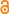Grzanna, J.; Lewerenz, H.-J.: Oscillations at the Si/electrolyte contact: Relation to Quantum Mechanics. Journal of Physics : Conference Series 574 (2015), p. 012024/1-4
10.1088/1742-6596/574/1/012024
Open Accesn VersionAbstract:
The basic process at the surface of the Si electrode is characterized by a cyclic oxidation of a thin silicon layer and the subsequent removal of the oxide by etching. Here, the oxide thickness evolves not uniformly due to cracks and nanopores. The mathematical model used to describe the phenomenon is based on a sequence of time dependent (oxide thickness) oscillator density functions that describes the passing of the (infinitesimal) oscillators through their minimum at each cycle. Two consecutive oscillator density functions are connected by a second order linear integral equation representing a Markov process. The kernel of the integral equation is a normalized Greens Function and represents the probability distribution for the periods of the oscillators during a cycle. Both, the oscillator density function and the two-dimensional probability density for the periods of the oscillators, define a random walk. A relation between the oscillator density functions and solutions of the Fokker-Planck equation can be constructed. This allows a connection of the oscillations, originally considered only for the description of a photo-electrochemical observation, to the Schrödinger equation. In addition, if the trajectory of a virtual particle, located at the silicon oxide electrode surface, is considered during one oscillatory cycle, then it can be shown that the displacement of the particle measured at the electrode surface performs a Brownian motion.